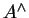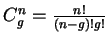Next: The Nothing Inside'' Test Up: Formula Processor Details Previous: Initial Graph Preprocessing

## Main Processing

The main parsing occurs once the initial graph has been created and preprocessed. The graph grammar is a set of rules which are templates describing the way various mathematical constructs are made.

The system checks all the rules in the grammar against the current formula graph, trying to find one that matches a subgraph in the current version of the formula's graph. If it is unable to find a rule that matches, it backtracks the parsing process to an earlier point that had more than one match and proceeds from there with an alternative choice. The backtracking is controlled by a priority queue using a simple heuristic. The heuristics will choose the graph with the smallest number of nodes. In the case of a tie, it chooses the search that has applied the largest number of rules so far.

The first rule that matches in this example is the superscript rule'', shown previously in Figure 3.1(b). This says that an element'' with another element'' to the top-right'' is a superscript. The A and B nodes are collapsed into a single node, their position and bounding box created from the two original nodes. Figure 3.6(d) shows the new graph. The data value for the new node is created by taking the data values of the original nodes, represented by A and B, and inserting them into the string{B}''.

The method used for searching for such a matching subgraph within the main graph is a brute force approach and is the main bottleneck of the parsing process. For every rule in the graph grammar, a search is done in current graph to see if it exists. To find a n node rule graph in an g node formula graph, all n node sub-graphs of the graph are generated, testing to see if any match the rule graph. The number of n node sub-graphs of a g node graph is. This search is repeated for every rule in the grammar. So, as the size of the formula graph grows, or the size and number of the rules in the grammar grow, the searching takes longer.

Bunke and Messmer  describe a method for speeding up attributed graph matching. This works by doing an initial pre-process of the rule graphs in the grammar and finding common substructures in them. They end up with a tree structure that describes how to build all the rule graphs progressively, starting from all the initial types of nodes.

The main graph is then searched for the rule graphs by, for each node in the graph, taking a node then testing all the rule graphs that could be built up starting with that type of node. This testing involves seeing, as the rule graphs are progressively built, whether they match the formula graph. Should they not match, the saving is made due to the fact that all the rule graphs that shared that non-matching sub-part are ruled out.

This technique was not used since, at the time the graph searching was implemented, it was not clear whether or not optimisations would be necessary. As this was only a prototype system, keeping the complexity of the system to a minimum was also desirable.

The next step applies the fraction'' rule. Figure(e) shows the new graph. Finally, the integral rule, shown in Figure 3.1(c), is applied. This gives the final graph, shown in Figure 3.6(e). From this point a number of productions are applied that change the node's data type from Element'' to Formula'', the parser's goal. It can be seen that as the parsing proceeds, the data'' value inside the nodes builds up to the final, in this case LATEX, representation of the formula.

If the parser gets to a point where it is unable to match any of the rules in the grammar to the current graph, it backtracks to an earlier point and tries alternative productions. Because of the current implementation of the graph processor, not having contextual information as Lavirotte and Pottier do, there are numerous cases where more than one rule can be applied to a given graph. These alternatives are tracked using a priority queue that prioritises based on the number of nodes that are in the graph and the current parse depth.

In determining the order in which to apply rules, a priority system can be used where each rule has a priority, either implicitly defined by its position in the grammar, or with an integer associated with each rule . A grammar can be constructed so that only a maximum of one rule in the grammar can be applied at a time. Lavirotte and Pottier  take this latter approach, describing a semi-automatic method for turning an ambiguous grammar to an unambiguous one by adding context rules'' that describe the conditions which must be true before a certain rule is applied. The system currently uses an implicit priority, based on the rule's position in the grammar, which means at times erroneous productions are made and the parser has to backtrack.

The only optimisation in the otherwise brute force graph matching is that before searching the graph to see if a rule matches, an initial test is done if all the node types required by the rule do exist somewhere within the graph.

Due to the implicit ordering of rules, a perfect'' parse almost always chooses the first rule that matches from the grammar every time. After finding that the slowest part of the parsing process was checking the rules in the grammar against the current formula graph, the system always initially follows the first match found in the grammar. When this approach finally runs out of choices, the system then backtracks and looks for further matches.

take this latter approach, describing a semi-automatic method for turning an ambiguous grammar to an unambiguous one by adding context rules'' that describe the conditions which must be true before a certain rule is applied. The system currently uses an implicit priority, based on the rule's position in the grammar, which means at times erroneous productions are made and the parser has to backtrack.

The only optimisation in the otherwise brute force graph matching is that before searching the graph to see if a rule matches, an initial test is done if all the node types required by the rule do exist somewhere within the graph.

Due to the implicit ordering of rules, a perfect'' parse almost always chooses the first rule that matches from the grammar every time. After finding that the slowest part of the parsing process was checking the rules in the grammar against the current formula graph, the system always initially follows the first match found in the grammar. When this approach finally runs out of choices, the system then backtracks and looks for further matches.Next: The Nothing Inside'' Test Up: Formula Processor Details Previous: Initial Graph Preprocessing
Steve Smithies
1999-11-13